## Introduction

ggplot() initializes a ggplot object. It can be used to declare the input data frame for a graphic and to specify the set of plot aesthetics intended to be common throughout all subsequent layers unless specifically overridden.

ggplot is a package for R. In Python, we will use plotnine, which is extremely similar to ggplot.

For R:

1. Download package ggplot that integrated in package tidyverse.

For Python:

1. Download Anaconda Environment, otherwise you will not have package pandas and numpy.
2. Download package plotnine through pip. If you never heard pip, click here.

### Understand ggplot

To understand the logic of ggplot, you’d better learn what is the principles of graphics.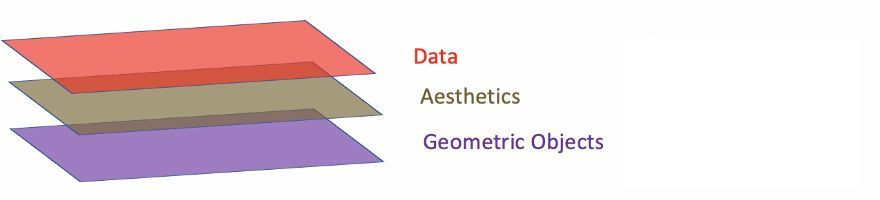1. Data: Data must be data.frame.
2. Aesthetics: Aesthetics is used to indicate x and y variables. It can also be used to control the color, the size or the shape of points, the height of bars, or etc.
3. Geometric Objects: A layer combines data, aesthetic mapping, a geom (geometric object), a stat (statistical transformation), and a position adjustment. Typically, you will create layers using a geom_ function, overriding the default position and stat if needed.

Basically:
Data: Assume your data are two points, for example, point $(0, 0)$ and $(1, 1)$. You need to tell the ggplot your data source.

Aesthetics: Then you need to define the x variables and y variables.

Geometric Objects: Tell ggplot what kind of plot - the object, you need. Let’s draw it on your paper.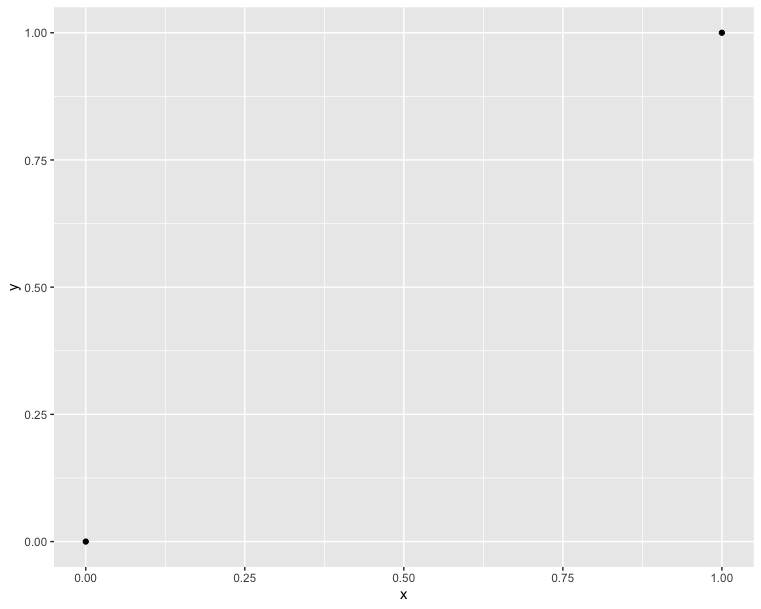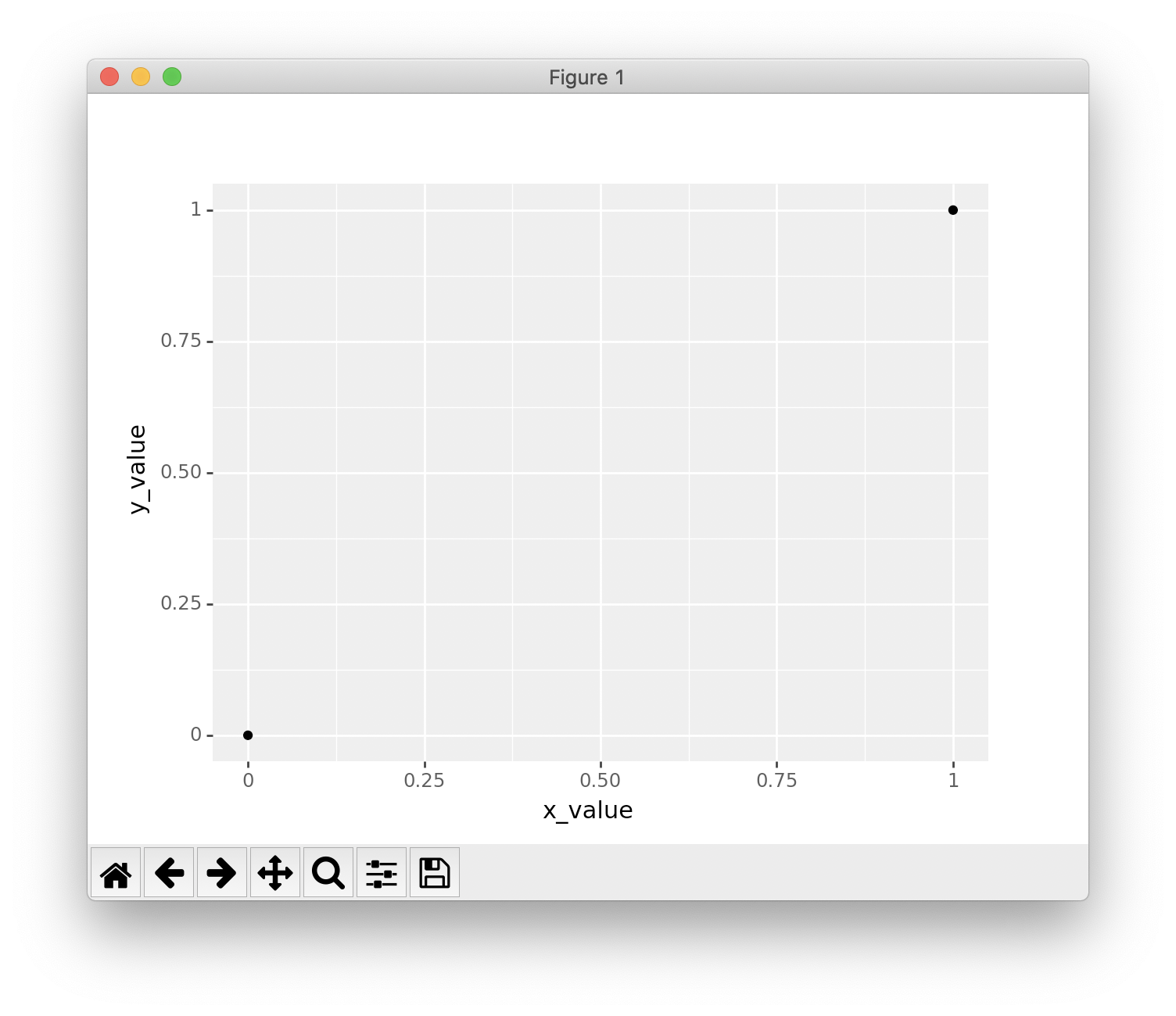Observe the difference of two part of code from R and Python. You must notice that Python need to create a dictionary first then convert it to data.frame. Because Python do not have data type named data.frame. You have to import package pandas then load data.frame.

Let’s put the main part of R code and Python code together, and find out the difference in Code.

In Python:

1. You must add an extra () to cover the whole ggplot expression.
2. You can declare data = data frame in R; but you cannot declare it in Python.
3. You must use '' or "" to wrap your parameters in the ggplot expression. But NOT for data frame.
4. You can put the + in the end or the beginning of each line.

In R:

1. You can omit data = in the first line.
2. You must put the + in the end of each line.

This time, we try to connect the two point. That is the simplest function - directly proportional function. And it is also the simplest plot - line chart.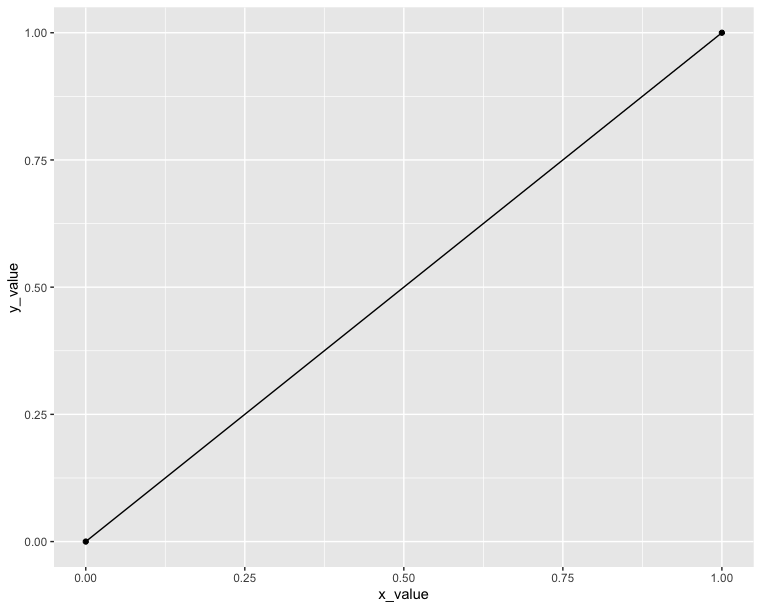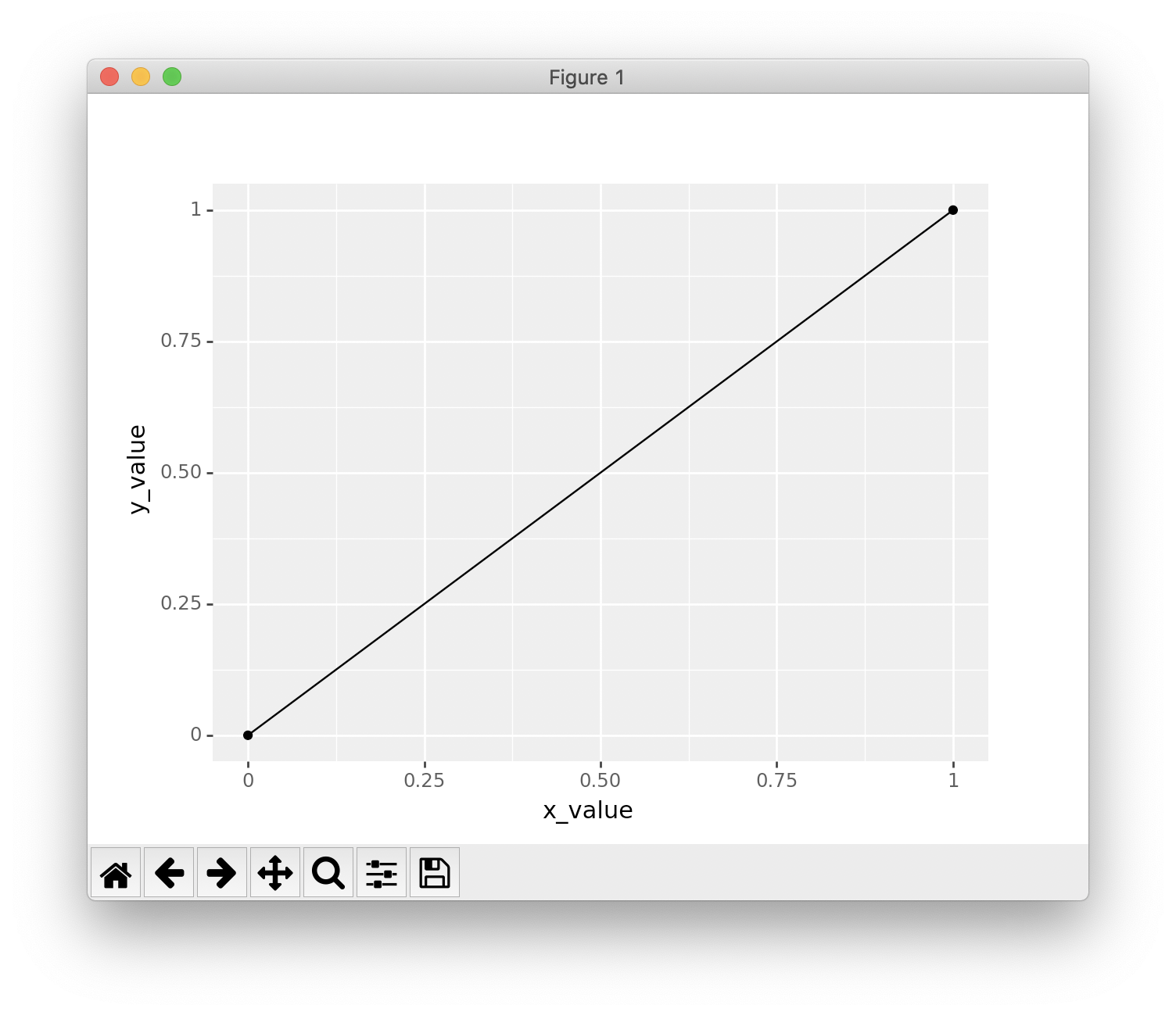### Explore more layers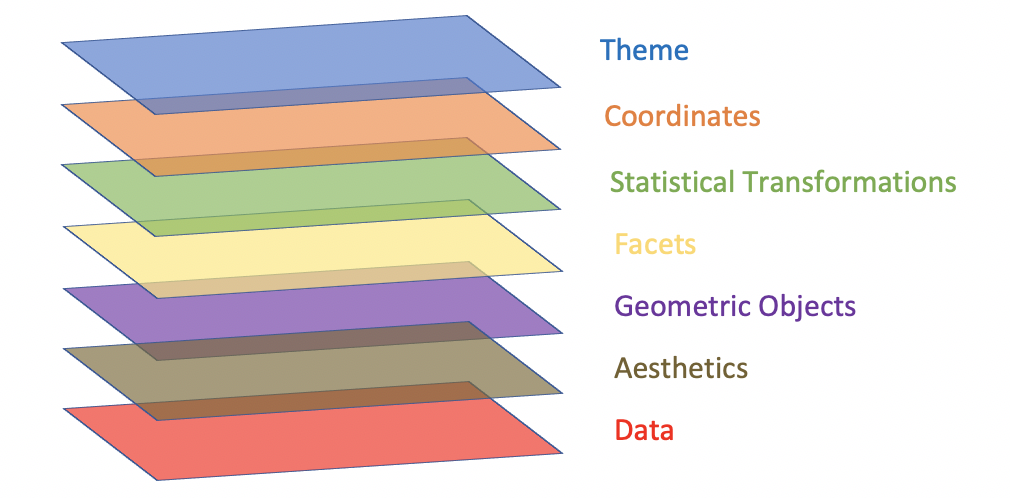Now we have understood the first three layers. Then let’s try to explore the rest four layers. If you cannot understand know, don’t worry. Just remember the concepts of layers is here.

See more detailed definition, click ggplot function reference.

From bottom to top:

1. Data: Data must be data.frame.
2. Aesthetics: Aesthetics is used to indicate x and y variables. It can also be used to control the color, the size or the shape of points, the height of bars, or etc.
3. Geometric Objects: A layer combines data, aesthetic mapping, a geom (geometric object), a stat (statistical transformation), and a position adjustment. Typically, you will create layers using a geom_ function, overriding the default position and stat if needed.
4. Facets: Facetting generates small multiples, each displaying a different subset of the data. Facets are an alternative to aesthetics for displaying additional discrete variables.
5. Statistical Transformations: A handful of layers are more easily specified with a stat_ function, drawing attention to the statistical transformation rather than the visual appearance. The computed variables can be mapped using stat().
6. Coordinates: The coordinate system determines how the x and y aesthetics combine to position elements in the plot. The default coordinate system is Cartesian (coord_cartesian()), which can be tweaked with coord_map(), coord_fixed(), coord_flip(), and coord_trans(), or completely replaced with coord_polar().
7. Themes: Themes control the display of all non-data elements of the plot. You can override all settings with a complete theme like theme_bw(), or choose to tweak individual settings by using theme() and the element_ functions. Use theme_set() to modify the active theme, affecting all future plots.